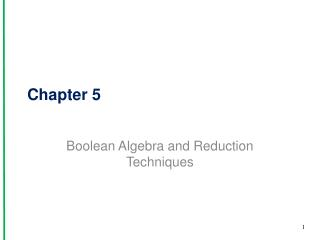DownloadDownload PresentationChapter 5

# Chapter 5

Télécharger la présentation## Chapter 5

- - - - - - - - - - - - - - - - - - - - - - - - - - - E N D - - - - - - - - - - - - - - - - - - - - - - - - - - -
##### Presentation Transcript

1. Chapter 5 Boolean Algebra and Reduction Techniques 1

2. 5-9 Karnaugh Mapping • Used to minimize the number of gates • Reduce circuit cost • Reduce physical size • Reduce gate failures • Requires SOP form • Karnaugh Mapping • Graphically shows output level for all possible input combinations • Moving from one cell to an adjacent cell, only one variable changes 31

3. Karnaugh Mapping • Steps for K-map reduction: • Transform the Boolean equation into SOP form • Fill in the appropriate cells of the K-map • Encircle adjacent cells in groups of 2, 4 or 8 • Adjacent means a side is touching, NOT diagonal. • Watch for the wraparound • Find each term of the final SOP equation by determining which variables remain the same within circles 33

4. Figure 5.86 Two-, three-, and four-variable Karnaugh maps.

5. Figure 5.87 Truth table and Karnaugh map of X =

6. Figure 5.88 Encircling adjacent cells in a Karnaugh map. These are the variables () that remain the same within each circle.

7. Discussion Point • Use a K-map to simplify the circuit.

8. 5-10 System Design Applications • Use Karnaugh Mapping to reduce equations • Use AND-OR-INVERT gates to implement logic

9. Figure 5.96 (a) Simplified equation derived from a Karnaugh map; (b) implementation of the odd-number decoder using an AOI.

10. Summary • Several logic gates can be connected together to form combinational logic. • There are several Boolean laws and rules that provide the means to form equivalent circuits. • Boolean algebra is used to reduce logic circuits to simpler equivalent circuits that function identically to the original circuit.

11. Summary • DeMorgan’s theorem is required in the reduction process whenever inversion bars cover more than one variable in the original Boolean equation. • NAND and NOR gates are sometimes referred to as universal gates, because they can be used to form any of the other gates.

12. Summary • AND-OR-INVERT (AOI) gates are often used to implement sum-of-products (SOP) equations. • Karnaugh mapping provides a systematic method of reducing logic circuits. • Combinational logic designs can be entered into a computer using schematic block design software or VHDL.

13. Summary • Using vectors in VHDL is a convenient way to group like signals together similar to an array. • Truth tables can be implemented in VHDL using vector signals with the selected signal assignment statement. • Quartus II can be used to determine the simplified equation of combinational circuits.